Olympiad Test: Completion Of Incomplete Pattern - 2

# Olympiad Test: Completion Of Incomplete Pattern - 2

Test Description

## 20 Questions MCQ Test Mathematical Olympiad Class 8 | Olympiad Test: Completion Of Incomplete Pattern - 2

Olympiad Test: Completion Of Incomplete Pattern - 2 for Class 8 2022 is part of Mathematical Olympiad Class 8 preparation. The Olympiad Test: Completion Of Incomplete Pattern - 2 questions and answers have been prepared according to the Class 8 exam syllabus.The Olympiad Test: Completion Of Incomplete Pattern - 2 MCQs are made for Class 8 2022 Exam. Find important definitions, questions, notes, meanings, examples, exercises, MCQs and online tests for Olympiad Test: Completion Of Incomplete Pattern - 2 below.
Solutions of Olympiad Test: Completion Of Incomplete Pattern - 2 questions in English are available as part of our Mathematical Olympiad Class 8 for Class 8 & Olympiad Test: Completion Of Incomplete Pattern - 2 solutions in Hindi for Mathematical Olympiad Class 8 course. Download more important topics, notes, lectures and mock test series for Class 8 Exam by signing up for free. Attempt Olympiad Test: Completion Of Incomplete Pattern - 2 | 20 questions in 40 minutes | Mock test for Class 8 preparation | Free important questions MCQ to study Mathematical Olympiad Class 8 for Class 8 Exam | Download free PDF with solutions
 1 Crore+ students have signed up on EduRev. Have you?
Olympiad Test: Completion Of Incomplete Pattern - 2 - Question 1

### Direction: In the following problems, select a figure from amongst the four options which when placed in the blank space of figure (X) would complete the pattern.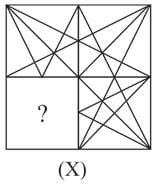Olympiad Test: Completion Of Incomplete Pattern - 2 - Question 2

### Direction: In the following problems, select a figure from amongst the four options which when placed in the blank space of figure (X) would complete the pattern.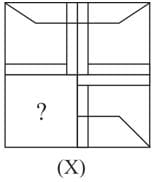Olympiad Test: Completion Of Incomplete Pattern - 2 - Question 3

### Direction: In the following problems, select a figure from amongst the four options which when placed in the blank space of figure (X) would complete the pattern.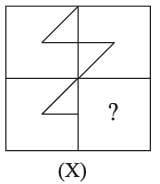Olympiad Test: Completion Of Incomplete Pattern - 2 - Question 4

Direction: In the following problems, select a figure from amongst the four options which when placed in the blank space of figure (X) would complete the pattern.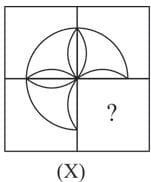Olympiad Test: Completion Of Incomplete Pattern - 2 - Question 5

Direction: In the following problems, select a figure from amongst the four options which when placed in the blank space of figure (X) would complete the pattern.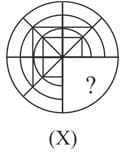Olympiad Test: Completion Of Incomplete Pattern - 2 - Question 6

Direction: In the following problems, select a figure from amongst the four options which when placed in the blank space of figure (X) would complete the pattern.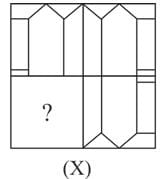Olympiad Test: Completion Of Incomplete Pattern - 2 - Question 7

Direction: In the following problems, select a figure from amongst the four options which when placed in the blank space of figure (X) would complete the pattern.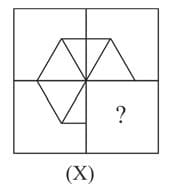Olympiad Test: Completion Of Incomplete Pattern - 2 - Question 8

Direction: In the following problems, select a figure from amongst the four options which when placed in the blank space of figure (X) would complete the pattern.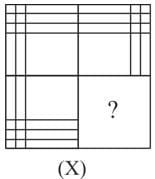Olympiad Test: Completion Of Incomplete Pattern - 2 - Question 9

Direction: In the following problems, select a figure from amongst the four options which when placed in the blank space of figure (X) would complete the pattern.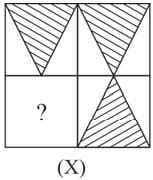Olympiad Test: Completion Of Incomplete Pattern - 2 - Question 10

Direction: In the following problems, select a figure from amongst the four options which when placed in the blank space of figure (X) would complete the pattern.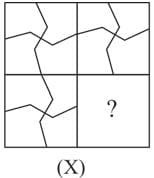Olympiad Test: Completion Of Incomplete Pattern - 2 - Question 11

Direction: In the following problems, select a figure from amongst the four options which when placed in the blank space of figure (X) would complete the pattern.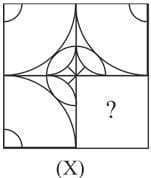Olympiad Test: Completion Of Incomplete Pattern - 2 - Question 12

Direction: In the following problems, select a figure from amongst the four options which when placed in the blank space of figure (X) would complete the pattern.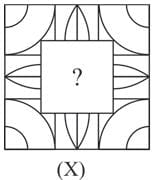Olympiad Test: Completion Of Incomplete Pattern - 2 - Question 13

Direction: In the following problems, select a figure from amongst the four options which when placed in the blank space of figure (X) would complete the pattern.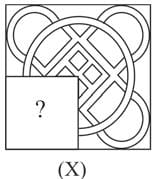Olympiad Test: Completion Of Incomplete Pattern - 2 - Question 14

Direction: In the following problems, select a figure from amongst the four options which when placed in the blank space of figure (X) would complete the pattern.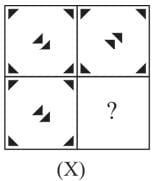Olympiad Test: Completion Of Incomplete Pattern - 2 - Question 15

Direction: In the following problems, select a figure from amongst the four options which when placed in the blank space of figure (X) would complete the pattern.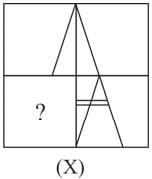Olympiad Test: Completion Of Incomplete Pattern - 2 - Question 16

Direction: In the following problems, select a figure from amongst the four options which when placed in the blank space of figure (X) would complete the pattern.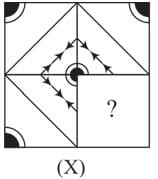Olympiad Test: Completion Of Incomplete Pattern - 2 - Question 17

Direction: In the following problems, select a figure from amongst the four options which when placed in the blank space of figure (X) would complete the pattern.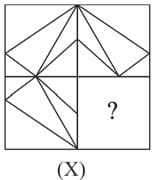Olympiad Test: Completion Of Incomplete Pattern - 2 - Question 18

Direction: In the following problems, select a figure from amongst the four options which when placed in the blank space of figure (X) would complete the pattern.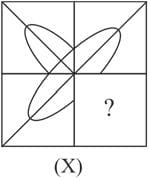Olympiad Test: Completion Of Incomplete Pattern - 2 - Question 19

Direction: In the following problems, select a figure from amongst the four options which when placed in the blank space of figure (X) would complete the pattern.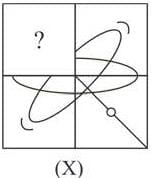Olympiad Test: Completion Of Incomplete Pattern - 2 - Question 20

Direction: In the following problems, select a figure from amongst the four options which when placed in the blank space of figure (X) would complete the pattern.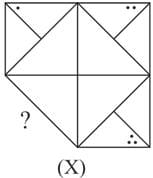## Mathematical Olympiad Class 8

53 tests
 Use Code STAYHOME200 and get INR 200 additional OFF Use Coupon Code
Information about Olympiad Test: Completion Of Incomplete Pattern - 2 Page
In this test you can find the Exam questions for Olympiad Test: Completion Of Incomplete Pattern - 2 solved & explained in the simplest way possible. Besides giving Questions and answers for Olympiad Test: Completion Of Incomplete Pattern - 2, EduRev gives you an ample number of Online tests for practice

53 tests